Question

# A particular dc motor has the following specifications: nominal voltage: 12V, no load RPM 19300, no...

A particular dc motor has the following specifications: nominal voltage: 12V, no load RPM 19300, no load current 1.4A, rated rpm 17100. Find torque, power, and linear and/or angular velocity.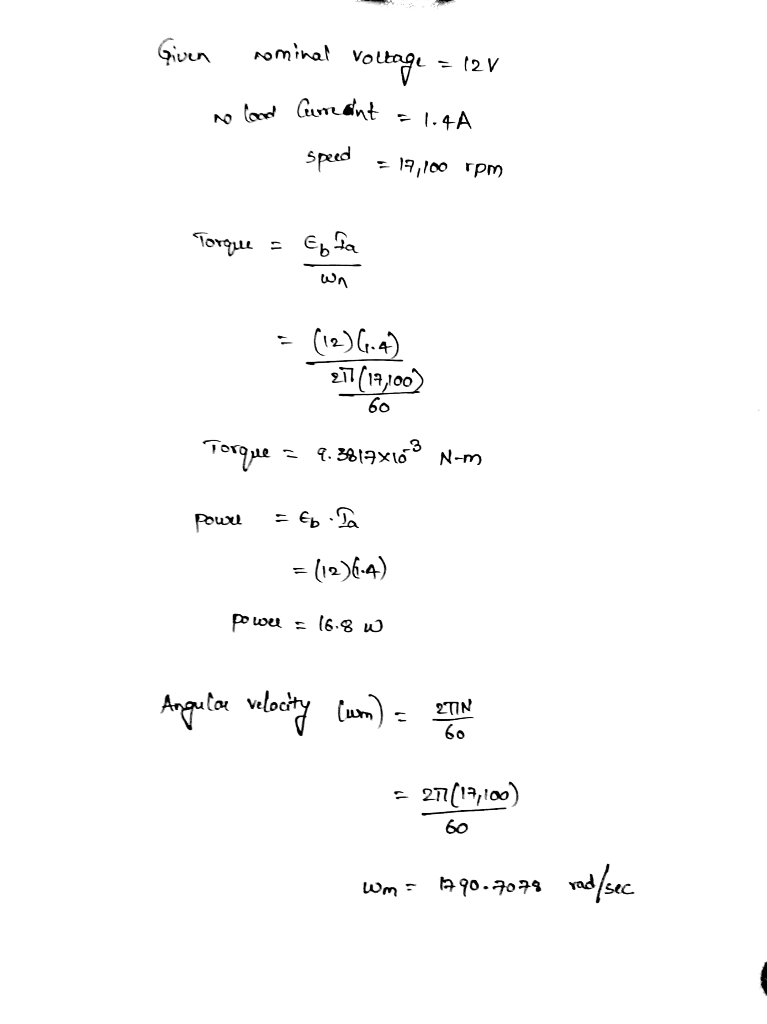#### Earn Coins

Coins can be redeemed for fabulous gifts.

Similar Homework Help Questions
• ### need the answer for the question no. 1 ENGR 113 Homework DC Motor Objectives Plot the...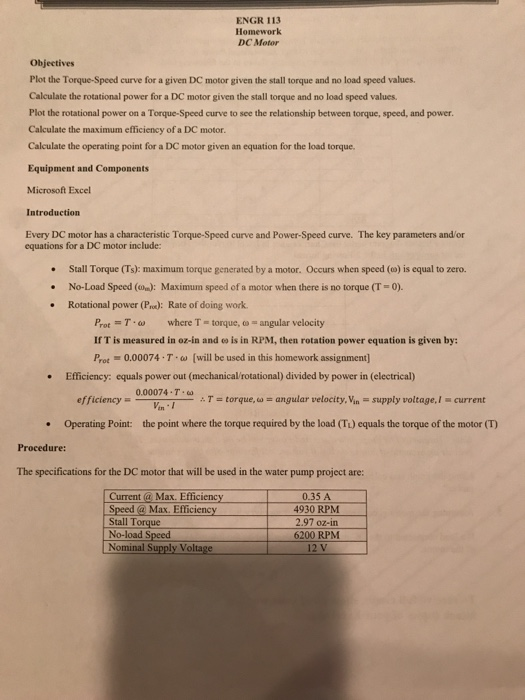need the answer for the question no. 1 ENGR 113 Homework DC Motor Objectives Plot the Torque-Speed curve for a given DC motor given the stall torque and no load speed values Calculate the rotational power for a DC motor given the stall torque and no load speed values Plot the rotational power on a Torque-Speed curve to see the relationship between torque, speed, and power Calculate the maximum efficiency of a DC motor. Calculate the operating point for a...

• ### If a manufacturer's specifications for a PM DC motor are as follows, what are the motor's...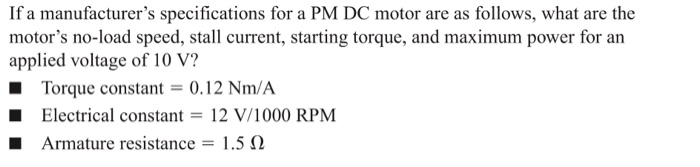If a manufacturer's specifications for a PM DC motor are as follows, what are the motor's no-load speed, stall current, starting torque, and maximum power for an applied voltage of 10 V? ■ Torque constant 0.12 Nm/A Electrical constant 12 V/1000 RPM Armature resistance-: 1.5 Ω

• ### Q5.8：The specifications of machine 5.8. For the machine in the previous question, calculate (a) its no-load speed (b)its rated efficiency-olcoxL-n/nN (c) the following when the speed is ze...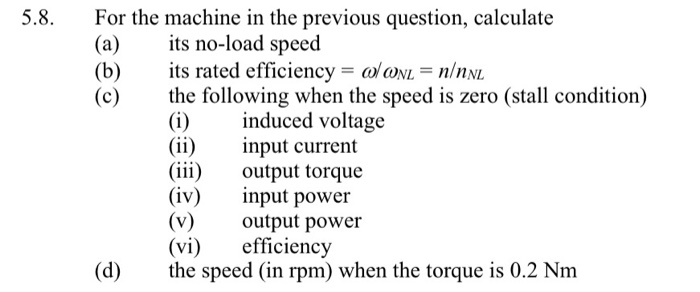Q5.8：The specifications of machine 5.8. For the machine in the previous question, calculate (a) its no-load speed (b)its rated efficiency-olcoxL-n/nN (c) the following when the speed is zero (stall condition) (i)induced voltage (ii) nput current (ii) output torque (iv) ut power (v)output power (vi) efficiency (d) he speed (in rpm) when the torque is 0.2 Nm 5 A DC machine has a no-load speed of 10,000 rpm and a rated speed of 8,000 rpm when operating with its rated supply...

• ### A separately excited DC motor has the following data P=32kW (rated power); Va=220V (rated voltage); Ia=171A...

A separately excited DC motor has the following data P=32kW (rated power); Va=220V (rated voltage); Ia=171A (rated curent); n=1000 rpm (rated speed); Ra = 0.096 ohm a.Calculate the armature voltage to reduce the speed to 900 rpm, assuming the load torque is kept constant? b.Calculate the resistance should be added to armature circuit to reduce the speed to 800 rpm, assuming the load torque is kept constant? c.Calculate the reduction of magnetic flux to reach the speed of 1100 rpm,...

• ### A 12-pole, brushless, DC motor has a no-load speed of 5000rpm and a stall torque of 50 in-oz when operating at rated voltage of 60V. Testing shows that no-load speed and stall torque vary linearly wi...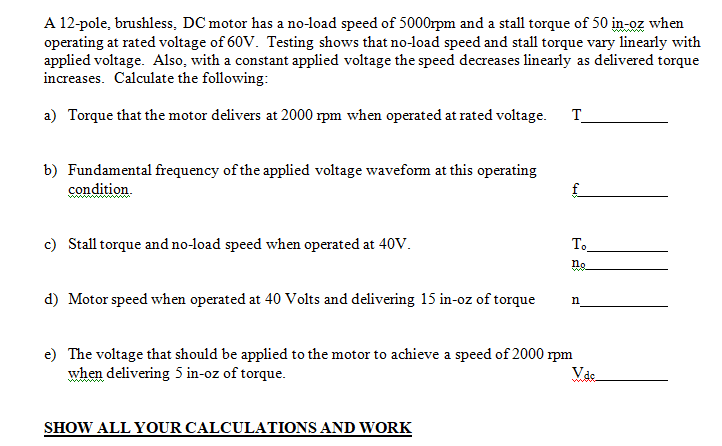A 12-pole, brushless, DC motor has a no-load speed of 5000rpm and a stall torque of 50 in-oz when operating at rated voltage of 60V. Testing shows that no-load speed and stall torque vary linearly with applied voltage. Also, with a constant applied voltage the speed decreases linearly as delivered torque increases. Calculate the following: Torque that the motor delivers at 2000 rpm when operated at rated voltage. T a) b) Fundamental frequency of the applied voltage waveform at this...

• ### Q1b) DC Machines [ 6 marks ] You are given a 24 V DC motor. When...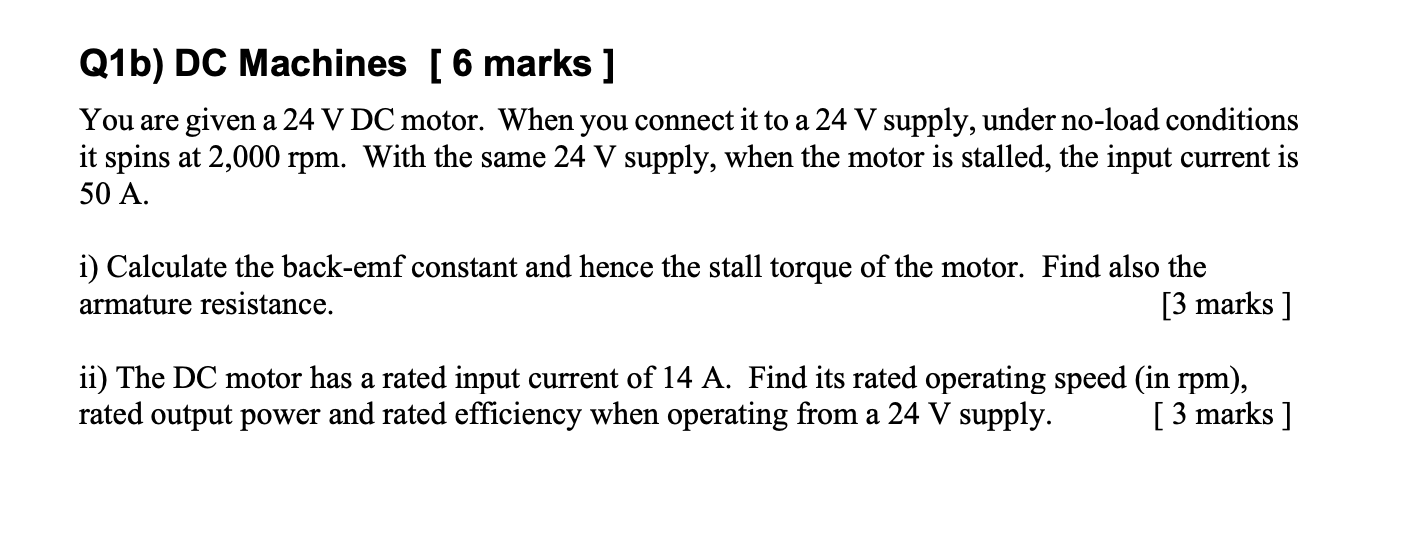Q1b) DC Machines [ 6 marks ] You are given a 24 V DC motor. When you connect it to a 24 V supply, under no-load conditions it spins at 2,000 rpm. With the same 24 V supply, when the motor is stalled, the input current is 50 A. i) Calculate the back-emf constant and hence the stall torque of the motor. Find also the armature resistance. [3 marks ] ii) The DC motor has a rated input current of...

• ### The three-phase wye-connected induction motor nameplate gives the following information: 400 V, 1755 rpm, 60 Hz....

The three-phase wye-connected induction motor nameplate gives the following information: 400 V, 1755 rpm, 60 Hz. Rotor speed at no-load is 1799 rpm. At rated voltage and frequency motor core losses are 400 W. At rated rotor speed, mechanical losses are 400 W. The available parameters of the motor conventional equivalent circuit (6-parameters IEEE model) are: R1 = 0.2 Ω, R’2 = 0.2 Ω, X1 = 0.5 Ω, X’2 = 0.2 Ω and Xφ = 20.0 Ω. In all calculations,...

• ### PROBLEM 2 (10 pts.) An Ideal DC motor has a no load (free running) speed of...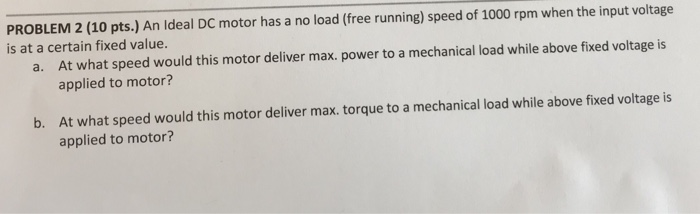PROBLEM 2 (10 pts.) An Ideal DC motor has a no load (free running) speed of 1000 rpm when the input voltage is at a certain fixed value. At what speed would this motor deliver max. power to a mechanical load while above fixed voltage is applied to motor? a. At what speed would this motor deliver max. torque to a mechanical load while above fixed voltage is applied to motor? b.

• ### (i) A 220 volts, 825 rpm, 125 A separately excited DC motor has an armature resistance...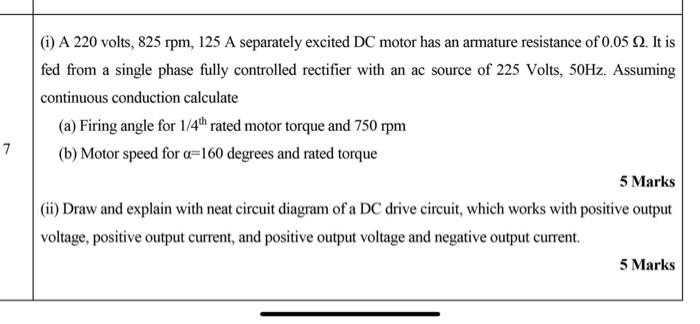(i) A 220 volts, 825 rpm, 125 A separately excited DC motor has an armature resistance of 0.05 2. It is fed from a single phase fully controlled rectifier with an ac source of 225 Volts, 50Hz. Assuming continuous conduction calculate (a) Firing angle for 1/4"hrated motor torque and 750 rpm (b) Motor speed for a=160 degrees and rated torque 5 Marks (ii) Draw and explain with neat circuit diagram of a DC drive circuit, which works with positive output...

• ### 3. A 20kW, 250V, 1000rpm shunt excited dc motor has armature and field resistances of 0.2...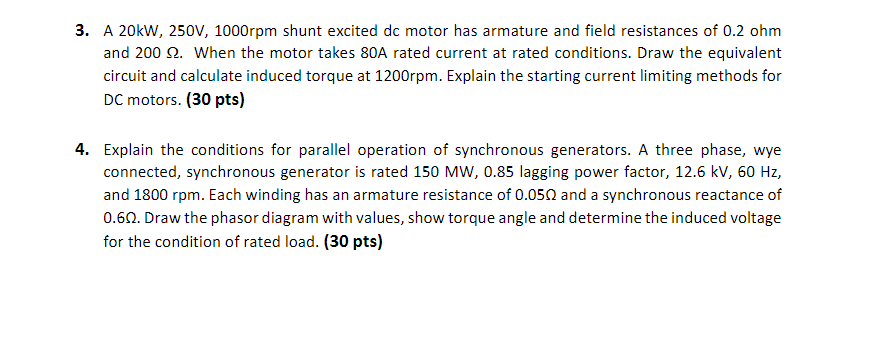3. A 20kW, 250V, 1000rpm shunt excited dc motor has armature and field resistances of 0.2 ohm and 2002. When the motor takes 80A rated current at rated conditions. Draw the equivalent circuit and calculate induced torque at 1200rpm. Explain the starting current limiting methods for DC motors. (30 pts) 4. Explain the conditions for parallel operation of synchronous generators. A three phase, wye connected, synchronous generator is rated 150 MW, 0.85 lagging power factor, 12.6 kV, 60 Hz, and...